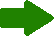# Highlights# CalendarOctober2018Mo. Tu. We. Th. Fr. Sa. Su. 1 2 3 4 5 6 7 8 9 10 11 12 13 14 15 16 17 18 19 20 21 22 23 24 25 26 27 28 29 30 31 1 2 3 4

# Finite-time average consensus protocols

Nowadays, several distributed estimation algorithms are based on the average consensus concept. Average consensus can be reached using a linear iterations scheme where each node repeatedly updates its value as a weighted linear combination of its own value and those of its neighbors; the main benefit of using a linear iterations scheme is that, at each time-step, each node only has to transmit a single value to each of its neighbors. Based on such a scheme, several algorithms have been proposed in the literature; however, in the majority of the proposed algorithms the weights are chosen so that all the nodes asymptotically converge to the same value. Sometimes, consensus can be embedded as a step of more sophisticated distributed; obviously, asymptotic convergence is not suitable for these kinds of distributed methods, and therefore it is interesting to address the question of exact consensus in finite-time. For time-invariant network topologies and in the perfect information exchange case, i.e., without channel noise nor quantization, we have shown that the finite-time average consensus problem can be solved as a matrix factorization problem with joint diagonalizable matrices depending on the graph Laplacian eigenvalues; moreover, the number of iterations is equal to the number of distinct nonzero eigenvalues of the graph Laplacian matrix. The design of such a protocol requires the knowledge of the Laplacian spectrum, which can be carried out in a distributed way. The matrix factorization problem is solved in a distributed way, in particular a learning method was proposed and the optimization problem was solved by means of distributed gradient backpropagation algorithms. The factor matrices are not necessarily symmetric and the number of these factor matrices is exactly equal to the diameter of the graph.

# Distributed graph discovery

We have studied the problem of estimating the eigenvalues of the Laplacian matrix associated with a graph modeling the interconnections between the nodes of a given network. Two approaches have been developed: for the first one, based on properties of the observability matrix, we have shown that Laplacian eigenvalues can be recovered by solving a local eigenvalue decomposition on an appropriately constructed matrix of observed data. Unlike FFT based methods recently proposed in the literature, in our method we are also able to estimate the multiplicities of the eigenvalues; however, this method is only applicable to networks having nodes with sufficient storage and computation capabilities, that is why we have proposed a second method requiring much less computation and storage capabilities. Based on a recent result showing that the average consensus matrix can be factored in D Laplacian based consensus matrices, where D stands for the number of nonzero distinct Laplacian eigenvalues, we have shown how carrying out such a factorization in a fully distributed way. The proposed solution results on a distributed solution of a constrained consensus problem. The availability of information on the communication topology of a wireless sensor network is essential for the design of the estimation algorithms. In the context of distributed self-organized sensor networks, there is no central unit with the knowledge of the network, and the agents must run some distributed networkdiscovery algorithms; this is particularly difficult in the case when the agents do not have or do not want to disclose their identifiers (id.s), either for technological reasons (in time-varying self-organized networks, assigning unique identifiers to agents is a challenge) or for privacy concerns. In a recent work it has been proposed an algorithm which allows each agent to find an estimate of the number of agents in the network, in an anonymous way; such an algorithm is based on the generation of pseudo-random numbers, on some consensus algorithms (for distributed computation either of average or of maximum), and on statistical inference. In our work, we show how the same algorithm, with some minor modifications, can provide more information: approximations of each node's eccentricity, of the graph diameter and of the graph radius. We study the quality of such approximations, providing tight bounds on the error.

# Observability in consensus networks

Studying the observability problem of a system consists in answering the question: is it possible, for a given node, to reconstruct the entire network state just from its own measurements and those of its neighbors? Studying observability for arbitrary graphs is particularly a tough task, therefore, studies are generally restricted to some families of graphs; for instance, recently, observability has been studied for paths and circular graphs where the study was carried out based on rules on number theory. Herein, we have considered families of graphs admitting an association scheme such that strongly regular graphs and distance regular graphs. The regularity properties of these kinds of graphs can particularly be useful for robustifying the network as for cryptographic systems. Based on the so-called Bose-Mesner algebra, we have stated observability conditions on consensus networks modeled with graphs modeled with strongly regular graphs and distance regular graphs; for this purpose, we have introduced the notion of local observability bipartite graph that allows characterizing the observability in consensus networks. We have shown that the observability condition is given by the nullity of the so-called "local bipartite observability graph;" when the nullity of the graph cannot be derived directly from the structure of the local bipartite observability graph, the rank of the associated bi-adjacency matrix allows evaluating the observability. The bi-adjacency matrix of the local bipartite observability graph must be full column rank for guaranteeing observability. From this general necessary and sufficient condition, we have deduced sufficient conditions for strongly regular graphs and distance regular graphs.

# Selected papersGo to the full list of publications